Get instant live expert help with Excel or Google Sheets“My Excelchat expert helped me in less than 20 minutes, saving me what would have been 5 hours of work!”

#### Post your problem and you’ll get expert help in seconds.

Your message must be at least 40 characters
Our professional experts are available now. Your privacy is guaranteed.

# Here is How to Use Excel’s MATCH Function

We can use excel MATCH FUNCTION to locate the position of a lookup value in a column, row, or table. Approximate and exact matching are supported by MATCH FUNCTION and wildcards (* ?) for partial matches. The steps below will walk through the process.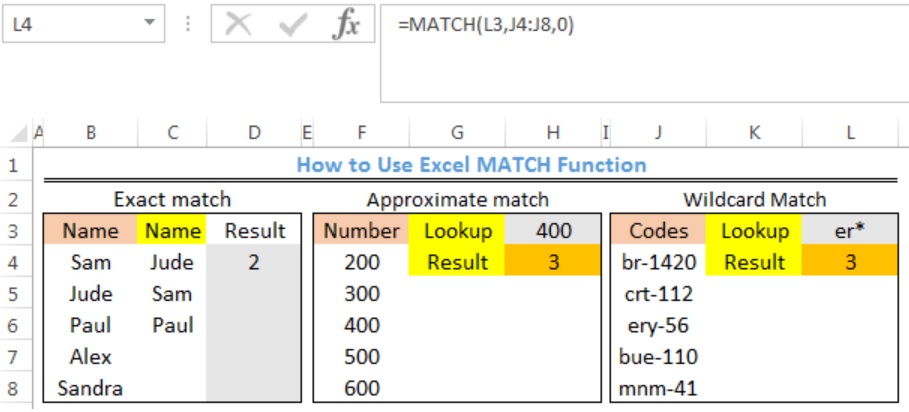Figure 1- How to Use Excel MATCH Function

## Syntax

`=MATCH(lookup_value,lookup_array,match_type)`

• lookup_value: This is the value to match in lookup_array.
• lookup_array – This is a range of cells or an array reference.
• match_type [optional]: How to match, specified as -1, 0, or 1. Default is 1.

## Formulas

Exact match: `=MATCH(C4,\$B\$4:\$B\$8,0)`

Approximate match: `=MATCH(H3,F4:F8,1) `

Wildcard match: `=MATCH(L3,J4:J8,0)`

## Setting up the Data

We will use the data in figure 2 to find an exact match for the names Jude, Sam, and Paul. We will also find the lookup result for 400 and er*.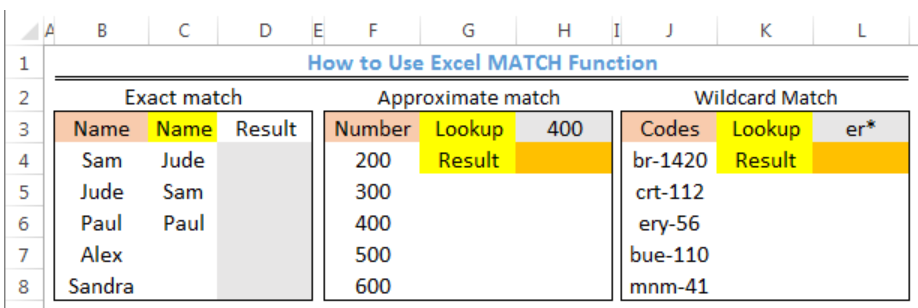Figure 2 – Setting up the Data

## Exact MATCH

If match_type is 0, the MATCH FUNCTION will return the first value that is exactly equal to the lookup_value.

• We will click on Cell D4
• We will insert the formula below into Cell D4
`=MATCH(C4,\$B\$4:\$B\$8,0)`
• We will press the enter key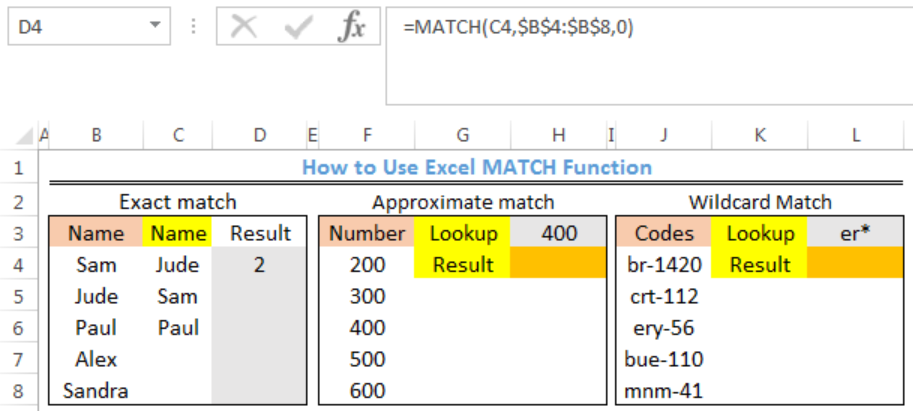Figure 3- Exact Match Result for Jude

• We will click on Cell D4 again
• We will double click on the fill handle tool which is the small plus sign you see at the bottom right of Cell D4. Select and drag down to copy the formula to Cell D6.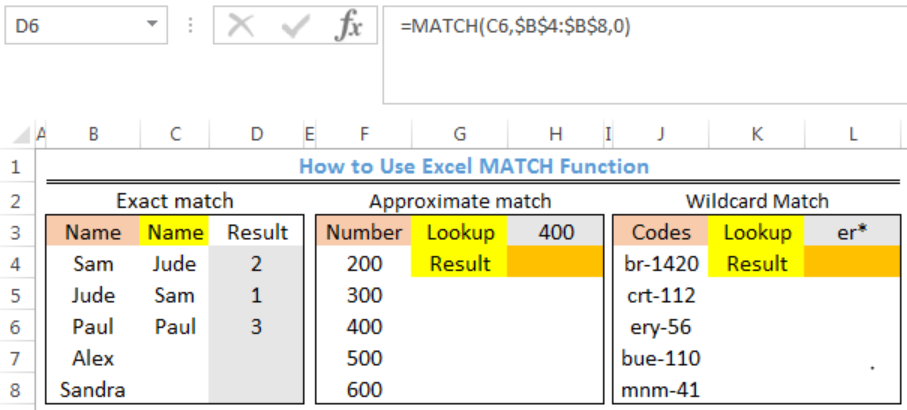Figure 4- Results for Exact Match

## Approximate MATCH

If match_type is 1, the MATCH function will find the largest value that is less than or equal to the lookup_value. Here, the lookup_array must be sorted in ascending order.

• We will click on Cell H4
• We will insert the formula below into Cell H4
`=MATCH(H3,F4:F8,1)`
• We will press the enter key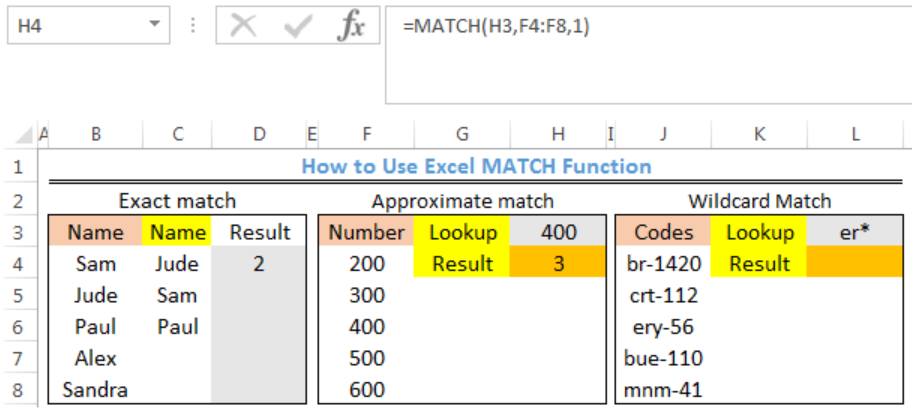Figure 5- Result for Approximate Match

## Wildcard Match

If match_type is 0, the MATCH function can find a match with wildcards. Here, the MATCH FUNCTION will find the code that begins with “er”

• We will click on Cell L4
• We will insert the formula below into Cell L4
`=MATCH(L3,J4:J8,0)`
• We will press the enter key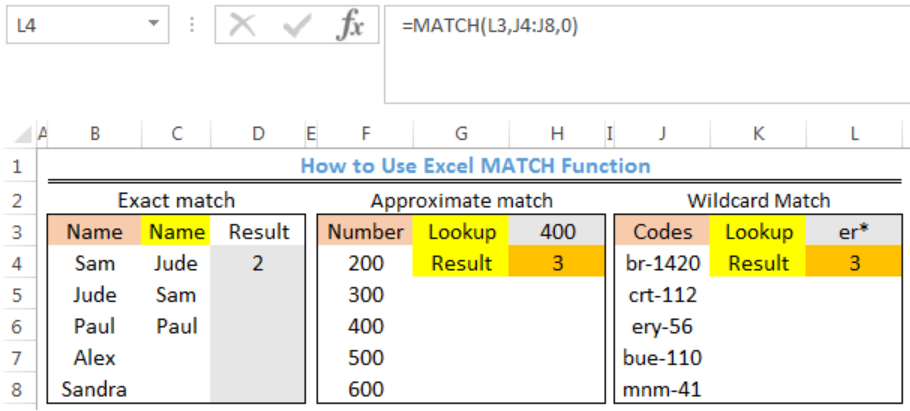Figure 6- Result for Wildcard Match

## Note

• If match_type is -1, the MATCH FUNCTION will find the smallest value that is greater than or equal to the lookup_value. The lookup_array must be sorted in descending order
• If we omit match_type, it is assumed to be 1
• MATCH FUNCTION is not case-sensitive.
• The MATCH FUNCTION returns #N/A error if no match is found
• If the match_type is 0 and lookup_value is text, then, wildcard characters like question mark (?) and asterisk (*) can be used in lookup_value

## Instant Connection to an Expert through our Excelchat Service

Most of the time, the problem you will need to solve will be more complex than a simple application of a formula or function. If you want to save hours of research and frustration, try our live Excelchat service! Our Excel Experts are available 24/7 to answer any Excel question you may have. We guarantee a connection within 30 seconds and a customized solution within 20 minutes.

Solution examplesNeed help with a formula, I'd like it to be something similar to '' =IF(AB2=AC2,"Matches","No Match") '' but instead of if they 100% match I want a 5% variable for example, if Cell A1 contains 12:00 and Cell B1 contains 11:59 I want it to class as matching.
Solved by Z. J. in 13 minsi want to hide a column but still use it in formula. is this even possible?
Solved by X. E. in 11 minsC5 contains 1625MPGW, F5 contains quantity. Need to search for MPGW and have quantity insert into column H5.
Solved by E. C. in 33 minsNeed help with lookup when you have multiple lookup values
Solved by V. J. in 20 minsI have a spread sheet with Items and sub Items. I would like to know if I can place a letter in another column it can get it to list the sub item number on another page in order
Solved by S. H. in 60 mins